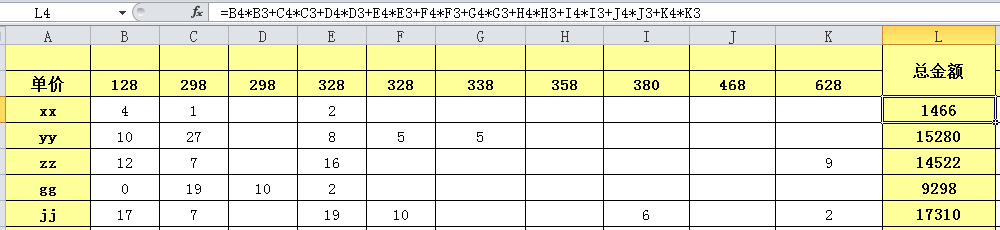# vi/vim的巧妙使用-数值加减,递增(转),序列等

## 1. 函数式, 数字加减

:s/替换字符串/\=函数式


:%s/\<id\>/\=line(".")                              " 将各行的 id 字符串替换为行号
:%s/^\<\w\+\>/\=(line(".")-10) .".". submatch(1)    " 将每行开头的单词替换为 (行号-10).单词 的格式，
" 如第11行的 word 替换成 1. word


# 1. 指南
## 1.0 资源
...
## 1.1 架构
...
# 2. 实践
...


%s/#\+\s*\zs\d*\ze\./\=submatch(0)+1/


## 2. 递增.方法一

#define BIT_MASK_1      (1 << 0)
#define BIT_MASK_2      (1 << 1)
#define BIT_MASK_3      (1 << 2)
#define BIT_MASK_4      (1 << 3)
#define BIT_MASK_5      (1 << 4)
#define BIT_MASK_6      (1 << 5)
#define BIT_MASK_7      (1 << 6)
#define BIT_MASK_8      (1 << 7)
#define BIT_MASK_9      (1 << 8)
#define BIT_MASK_10      (1 << 9)
#define BIT_MASK_11      (1 << 10)
#define BIT_MASK_12      (1 << 11)
#define BIT_MASK_13      (1 << 12)
#define BIT_MASK_14      (1 << 13)
#define BIT_MASK_15      (1 << 14)
#define BIT_MASK_16      (1 << 15)
#define BIT_MASK_17      (1 << 16)
#define BIT_MASK_18      (1 << 17)
#define BIT_MASK_19      (1 << 18)
#define BIT_MASK_20      (1 << 19)
#define BIT_MASK_21      (1 << 20)
#define BIT_MASK_22      (1 << 21)
#define BIT_MASK_23      (1 << 22)
#define BIT_MASK_24      (1 << 23)
#define BIT_MASK_25      (1 << 24)
#define BIT_MASK_26      (1 << 25)
#define BIT_MASK_27      (1 << 26)
#define BIT_MASK_28      (1 << 27)
#define BIT_MASK_29      (1 << 28)
#define BIT_MASK_30      (1 << 29)
#define BIT_MASK_31      (1 << 30)
#define BIT_MASK_32      (1 << 31)


#define BIT_MASK_1      (1 << 0)


## 2.1 命令

:'<,'>s/BIT_MASK_\zs\d*\ze/\=line(".") - line("'<") + 1
:'<,'>s/\zs\d*\ze)$/\=line(".")-line("'<") 【或】 :%s/BIT_MASK_\zs\d*\ze/\=line(".") - line("'<") + 1 :%s/\zs\d*\ze)$/\=line(".")-line("'<")


## 2.2 解析

:'<,'>s/BIT_MASK_\zs\d*\ze/\=line(".") - line("'<") + 1


'<,'>        我们所选中的区域 (:help '<，:help '> )
s            在选中的区域中进行替换 (:help :s )
\zs          指明匹配由此开始 (:help /\zs )
\d*          查找任意位数的数字 (:help /\d )
\ze          指明匹配到此为止 (:help /\ze )
\=           指明后面是一个表达式 (:help :s\= )
line(".")    当前光标所在行的行号 (:help line() )
line("'<")   我们所选区域中第一行的行号 (:help line() )
'<和'>是我们使用了"v"，"V"命令选中一个visual区域后，VIM设置的标记，分别用来标识visual区域的开始和结束。


## 2.3 Excel第3行为单价的求和示例

• 需求

Execl表格第L列 = SUM(单价<单价> * 数量<第N行>)，例如

L4 = B4*B3+C4*C3+D4*D3+E4*E3+F4*F3+G4*G3+H4*H3+I4*I3+J4*J3+K4*K3


并且，第L列有非常多的行• 问题

填写好Excel表格中的第L4列，然而，这时不能使用下拉填充的方式（L5会变成B5*B4+C5*C4+…）。这个问题可以用以上方法解决。

• 步骤一，用vim复制粘贴第L列N行（比如50行），如下：

=B4*B3+C4*C3+D4*D3+E4*E3+F4*F3+G4*G3+H4*H3+I4*I3+J4*J3+K4*K3
...
=B4*B3+C4*C3+D4*D3+E4*E3+F4*F3+G4*G3+H4*H3+I4*I3+J4*J3+K4*K3

• 步骤二，多执行几次如下命令就可以了

%s/[^0123456789]\+\zs4\ze\*/\=line(".")+3/
# 或
%s/[A-Z]\+\zs4\ze\*/\=line(".")+3/

• 步骤三，将结果拷贝粘贴到excel，完成！

## 3. 递增: 方法二

UniqueID2       = lview.focusedItem.subItems.opIndex(0).text;
Parent          = lview.focusedItem.subItems.opIndex(0).text;
Children        = lview.focusedItem.subItems.opIndex(0).text;
txtCust.text    = lview.focusedItem.subItems.opIndex(2).text;
txtProj.text    = lview.focusedItem.subItems.opIndex(3).text;
txtbDate.text   = lview.focusedItem.subItems.opIndex(4).text;
txtdDate.text   = lview.focusedItem.subItems.opIndex(5).text;
txteDate.text   = lview.focusedItem.subItems.opIndex(6).text;
txtPM.text      = lview.focusedItem.subItems.opIndex(7).text;
txtLang.text    = lview.focusedItem.subItems.opIndex(8).text;
txtVendor.text  = lview.focusedItem.subItems.opIndex(9).text;
txtInvoice.text = lview.focusedItem.subItems.opIndex(10).text;
txtPMFund.text  = lview.focusedItem.subItems.opIndex(11).text;
txtProjFund.text= lview.focusedItem.subItems.opIndex(12).text;
txtA_No.text    = lview.focusedItem.subItems.opIndex(13).text;
txtNotes.text   = lview.focusedItem.subItems.opIndex(14).text;
txtStatus.text  = lview.focusedItem.subItems.opIndex(15).text;


UniqueID2       = lview.focusedItem.subItems.opIndex(0).text;
Parent          = lview.focusedItem.subItems.opIndex(1).text;
Children        = lview.focusedItem.subItems.opIndex(2).text;
......


:let n=0 | g/opIndex(\zs\d\+/s//\=n/|let n+=1


let          为变量赋值 (:help let )
|            用来分隔不同的命令 (:help :bar )
g            在匹配后面模式的行中执行指定的ex命令 (:help :g )
\zs          指明匹配由此开始 (:help /\zs )
\d\+         查找1个或多个数字 (:help /\d )
s            在选中的区域中进行替换 (:help :s )
\=           指明后面是一个表达式 (:help :s\= )


给变量n赋值为0；



http://vim.sourceforge.net/scripts/script.php?script_id=670

http://mysite.verizon.net/astronaut/vim/index.html#VISINCR


## 4.1 [技巧一] 统计文章字数

:%s/\w//gn


%s            在整个文件中替换 (:help :s )
\w           匹配一个字 (word) (:help /\w )
g           替换行内所有出现的匹配 (:help :s_flags)
n           只报告匹配的数目，并不真正进行替换(:help :s_flags)


## 4.2 [技巧二] 将一串十进制数字转换为16进制数字

:%s/\d\+/\=printf("%X", submatch(0))/g


%s            在整个文件中替换 (:help :s )
\d\+            匹配一个或多个数字 (:help /\d  :help /\+ )
\=           使用表达式的结果进行替换 (:help /\w )
printf        按指定格式输出 (:help printf() )
submatch()    返回:s命令中的指定匹配字符串 (:help submatch() )
g           替换行内所有出现的匹配 (:help :s_flags)



# 5. 数字序列(range函数)

5
6
7
...
100


:s/^/\=range(5, 100)


:s/^/\=range(5, 100, 2)